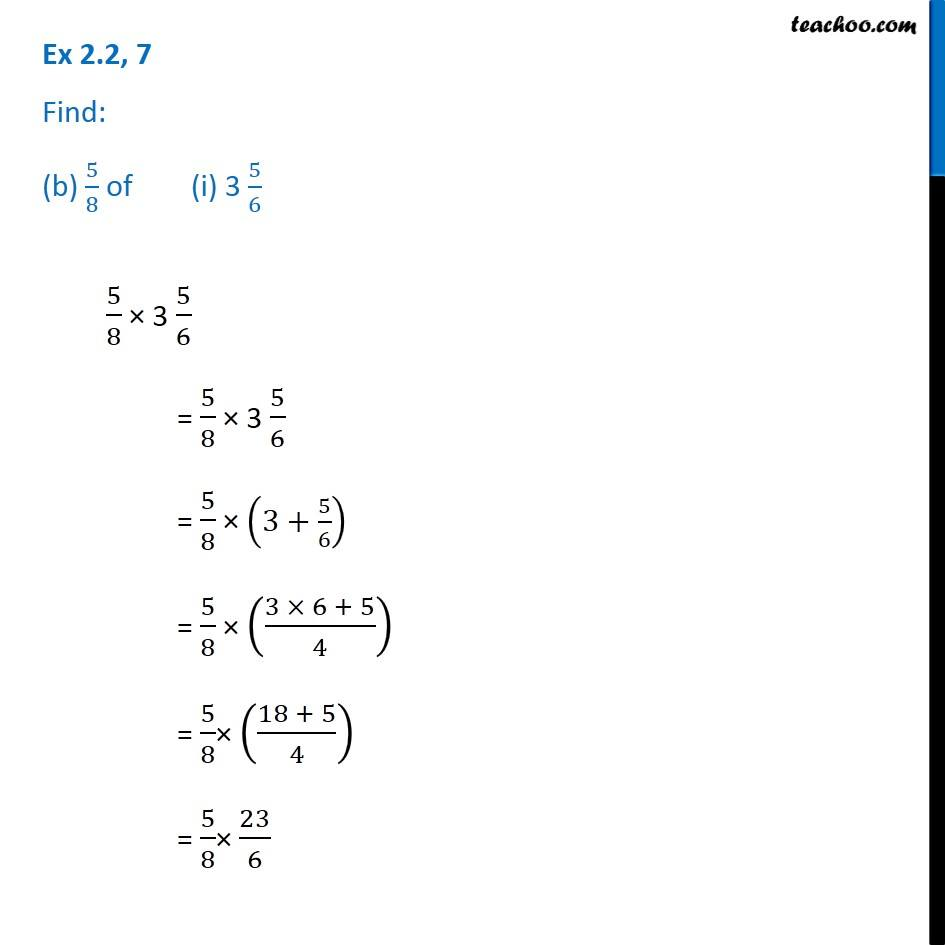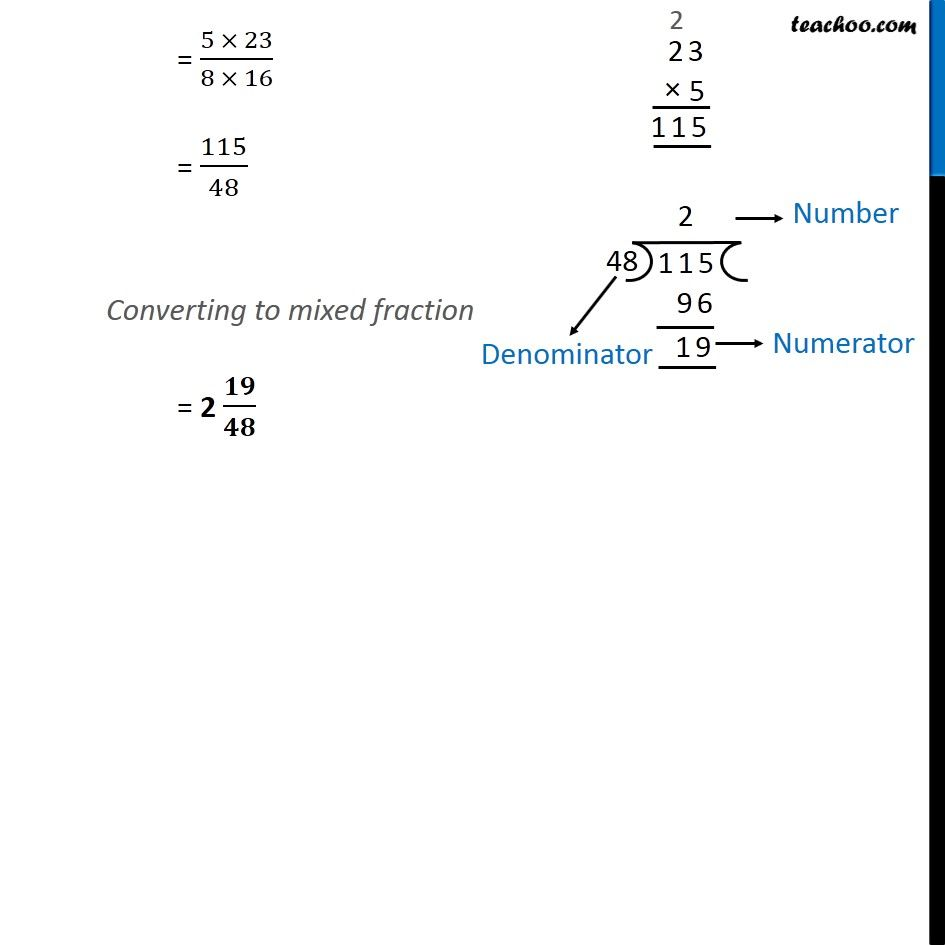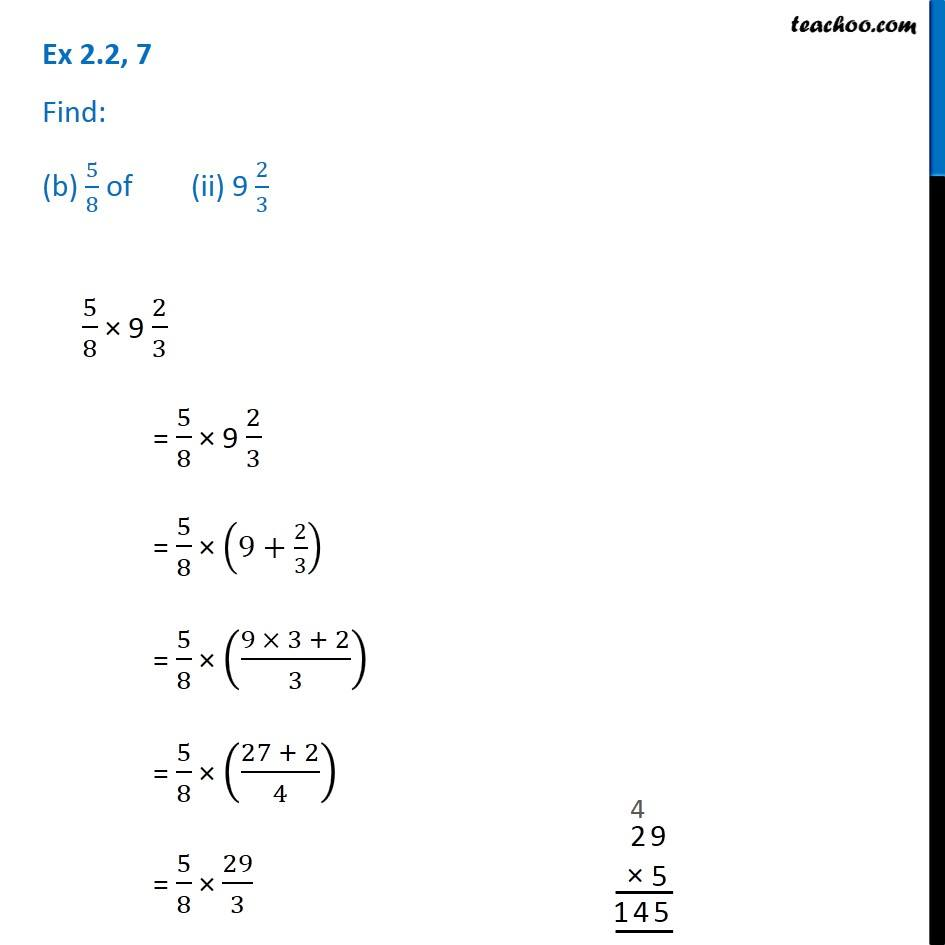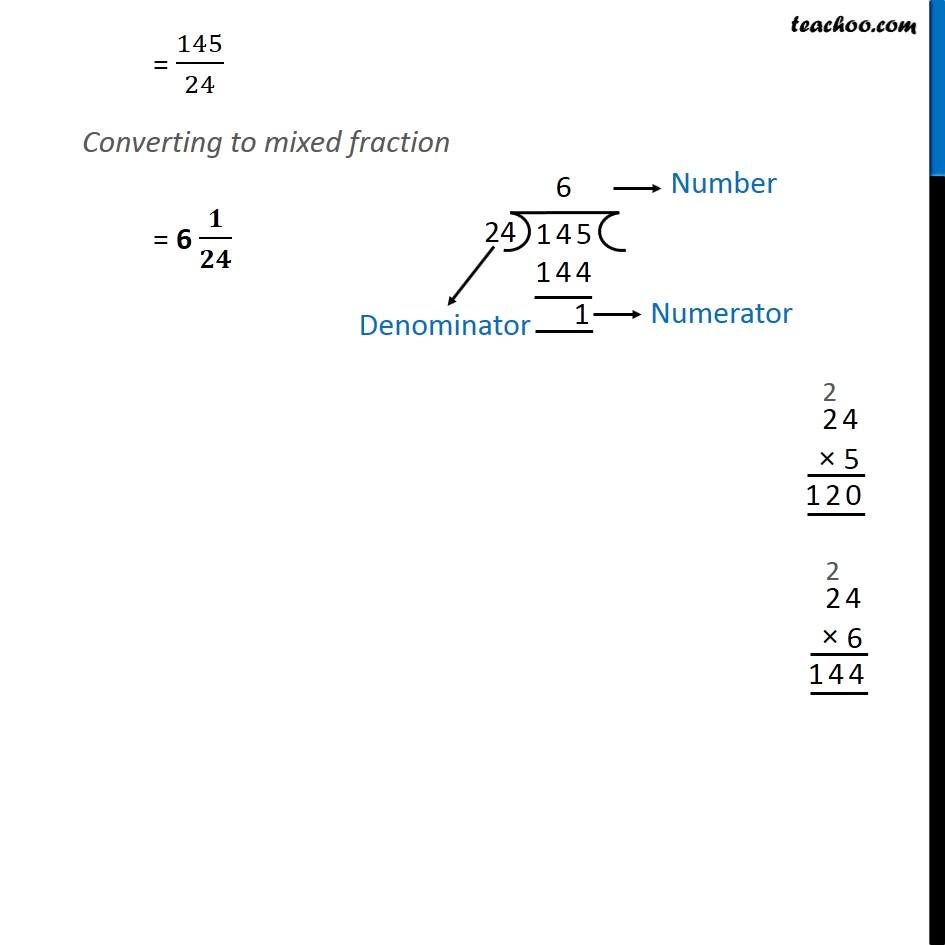Ex 2.2

Chapter 2 Class 7 Fractions and Decimals
Serial order wiseGet live Maths 1-on-1 Classs - Class 6 to 12

### Transcript

Ex 2.2, 7 Find: (b) 5/8 of (i) 3 5/65/8 × 3 5/6 = 5/8 × 3 5/6 = 5/8 × (3+5/6) = 5/8 × ((3 × 6 + 5)/4) = 5/8× ((18 + 5)/4) = 5/8× 23/6 = (5 × 23)/(8 × 16) = 115/48 Converting to mixed fraction = 2 𝟏𝟗/𝟒𝟖 Ex 2.2, 7 Find: (b) 5/8 of (ii) 9 2/35/8 × 9 2/3 = 5/8 × 9 2/3 = 5/8 × (9+2/3) = 5/8 × ((9 × 3 + 2)/3) = 5/8 × ((27 + 2)/4) = 5/8 × 29/3 = 145/24 Converting to mixed fraction = 6 𝟏/𝟐𝟒